Hello everyone!,
I am happy to announce the Math Olympiad Contest which only allows High School Math problems. I am organizing this contest so as to get exposure to various topics and tricks to solve hard problems. The rules are as follows:

1. Suppose problem $i$ is posted. The person who solves problem $i$ and posts the solution can publish problem $i+1$.
2. This will continue until the problem posted is not answered within 6 hours. If a solution is not posted, the problem maker themself will post a solution to the problem, and then pose a new problem.If the solution of a problem is not posted by the problem-poster within 10hrs.,then his/her marks will be deducted by 2.
3. I request that problem-posters contribute problems that are solvable and do not post problems that will demotivate others.
4. The contest will end once 20 problems have been posed and answered (problems not solved within 6 hours don't count toward this).
5. Problem posters should know the solution of their posted problem in advance.
6. If the new problem is not posted in 15 minutes of answering question $i$, ANYBODY can post question $i+1$.
7. Each problem will be designated a number of points between 1 and 5, decided by the problem poster. Please include this value in the statement of the problem.
8. I request all entries to be honest and fair. Do not check for the solution on the internet or copy-paste the solution.
9. I would also request that you re-share this note so that other members can learn about and join this contest.
10. If someone has posted a solution to a problem and if the problem-poster is not online,even others can post their solution till the problem-poster decides whose is the correct solution.The one who gives the fastest and correct solution gets the points.
11. The solutions posted after 6hrs.[i.e the time limit of a problem] will be not be considered.

Points:

1 . Kaustubh Miglani : 9 marks

2 . Ayush Rai : 7 marks

3 . Abhishek Alva,Svatejas Shivakumar,Archit Agrawal,Govind Ramesh : 3 marks

4 . Prakhar Bindal : 1 mark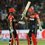Note by Ayush G Rai
4 years, 9 months ago

This discussion board is a place to discuss our Daily Challenges and the math and science related to those challenges. Explanations are more than just a solution — they should explain the steps and thinking strategies that you used to obtain the solution. Comments should further the discussion of math and science.

When posting on Brilliant:

• Use the emojis to react to an explanation, whether you're congratulating a job well done , or just really confused .
• Ask specific questions about the challenge or the steps in somebody's explanation. Well-posed questions can add a lot to the discussion, but posting "I don't understand!" doesn't help anyone.
• Try to contribute something new to the discussion, whether it is an extension, generalization or other idea related to the challenge.

MarkdownAppears as
*italics* or _italics_ italics
**bold** or __bold__ bold
- bulleted- list
• bulleted
• list
1. numbered2. list
1. numbered
2. list
Note: you must add a full line of space before and after lists for them to show up correctly
paragraph 1paragraph 2

paragraph 1

paragraph 2

[example link](https://brilliant.org)example link
> This is a quote
This is a quote
    # I indented these lines
# 4 spaces, and now they show
# up as a code block.

print "hello world"
# I indented these lines
# 4 spaces, and now they show
# up as a code block.

print "hello world"
MathAppears as
Remember to wrap math in $$ ... $$ or $ ... $ to ensure proper formatting.
2 \times 3 $2 \times 3$
2^{34} $2^{34}$
a_{i-1} $a_{i-1}$
\frac{2}{3} $\frac{2}{3}$
\sqrt{2} $\sqrt{2}$
\sum_{i=1}^3 $\sum_{i=1}^3$
\sin \theta $\sin \theta$
\boxed{123} $\boxed{123}$

Sort by:

Problem 2 , 3 marks

Prove that $ab=gcd×lcm$ of $a$ and $b$

- 4 years, 9 months ago

Problem 16:[1 marks] Find area of the graph [|(3x+4y)/5|]+[|4y-3x|/5]=3. Where [ ] denote greatest integer.

- 4 years, 9 months ago

- 4 years, 9 months ago

Archit wont be more in the contest I suppose because of your foolish attentions and such rules

- 4 years, 9 months ago

Attention:The actual problem 16 is not this.

- 4 years, 9 months ago

Problem 19 : [4 marks]
$\triangle ABC$ has sides $AB=168,BC=156,$ and $CA=180.$It is inscribed in a circle,which has center $O.$Let $M$ be the midpoint of $AB,$let $B'$ be the point on the circle diametrically opposite $B,$and let $X$ be the intersection of $AO$ and $MB'.$Find the length of $AX.$

- 4 years, 9 months ago- 4 years, 9 months ago

You too used a formula for the circumradius of the triangle

- 4 years, 8 months ago

Problem 1:[3 marks]$ABC$ and $DBC$ are two equilateral triangles on the same base $BC$.A point $P$ is taken on the circle with center $D$ and radius as $BD$.Show that $PA,PB$ and $PC$ are the sides of a right triangle where $P$ does not coincide with the points $B$ and $C$ on the circle.

- 4 years, 9 months ago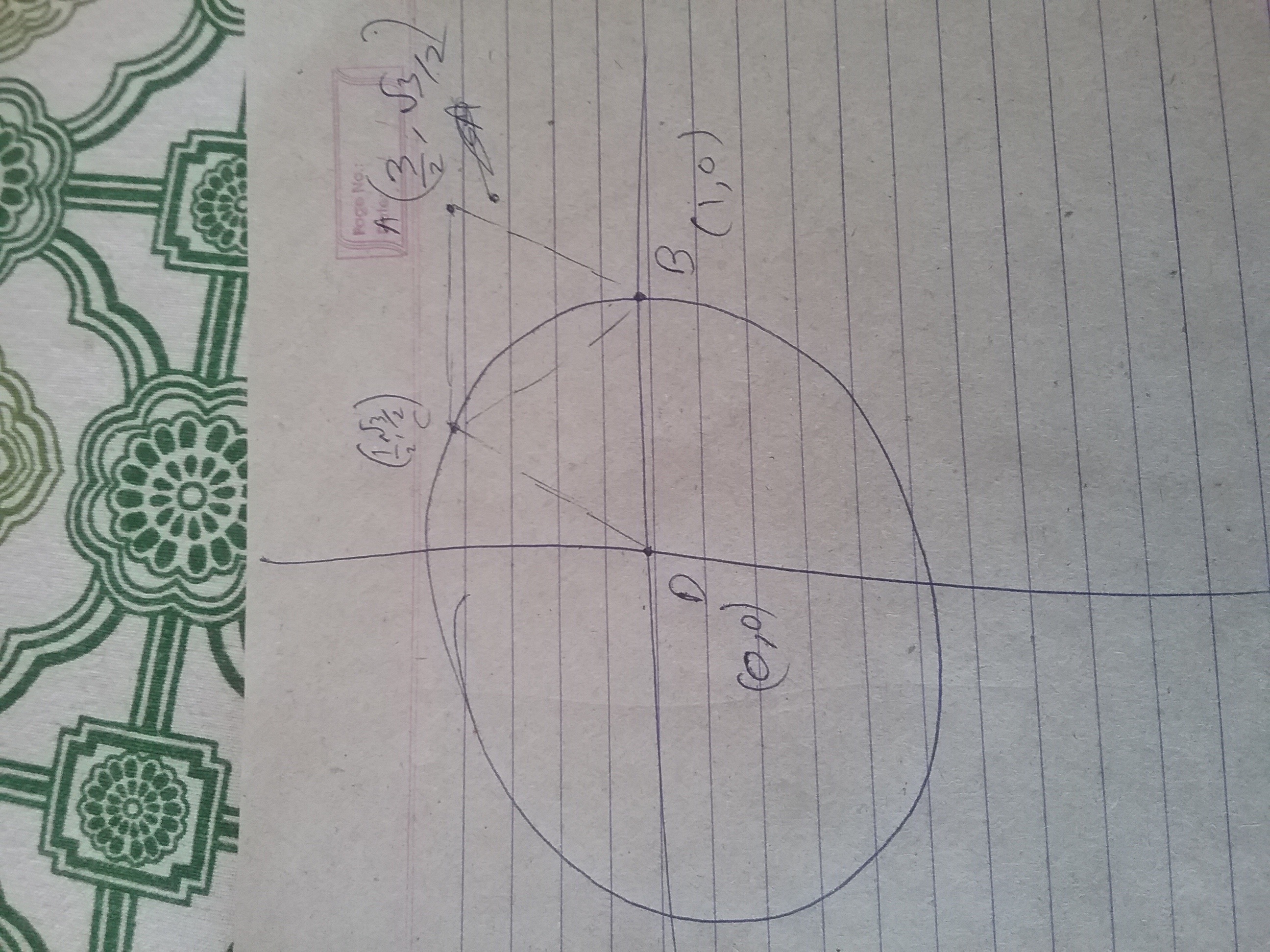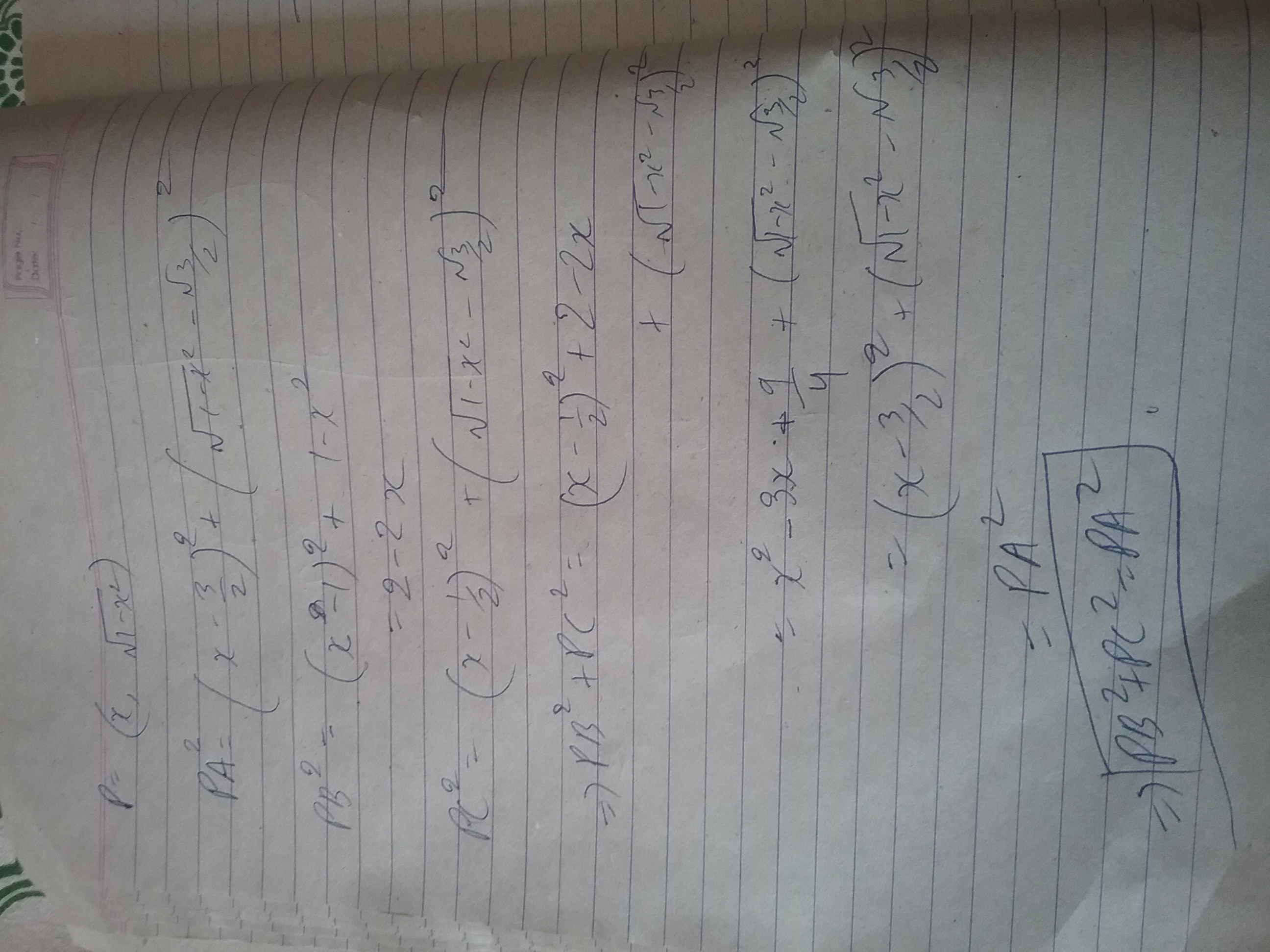- 4 years, 9 months ago

Good solution..+1,you are correct.you can proceed with the next problem

- 4 years, 9 months ago

here in the daigram it ab need not be the tangent

- 4 years, 9 months ago

Actually not need not, its CAN not as angle ABD is 120 degree and for tangent angle is 90 degree!

- 4 years, 9 months ago

yes u are right

- 4 years, 9 months ago

[Problem 2, 3 marks]

Prove that $ab=gcd (a,b)×lcm (a,b)$

- 4 years, 9 months ago

the answer lies in the defination of hcf and lcm.we can represent it as a venn daigram.the elements which are perfectly common are taken out which is the hcf and what remains behind are the elements which not perfectly common which is the lcm . when we do the union of the set hcf and lcm we get the original set which the union of the 2 numbers

- 4 years, 9 months ago

Wrong mathematical proof

- 4 years, 9 months ago

i can represent it using venn daigrams or sets.

- 4 years, 9 months ago

if u are still not convienced then lcm and hcf are like we are taking some elements from the product of the 2 set such that the all the elements are utilized.so when we unite both the lcm and the hcf we get the number back.the proof of this is the defination itself

- 4 years, 9 months ago

Yes you were writing exact answer, but in a theoritical way. So I can award only 2 points to you. And Ayush gets 3 points

- 4 years, 9 months ago

Case 1:When $a$ and $b$ are co-prime to each other
Since $a$ and $b$ are co-prime,the G.C.D. is $1.$The $L.C.M.$ will be $a\times b$ because $a$ and $b$ should divide the $L.C.M.$So since $a$ and $b$ do not have any common factors,the $L.C.M$ should be the product of $a$ and $b.$Therefore $ab=1\times(a\times b).ab=ab.$Hence proved.
Case 2:When a and b are not co-prime to each other
Let the $G.C.D$ be $k$ of $a$ and $b.$Well,$a\times b$ will contain $k$ since it is the common divisor of $a$ and $b.$So let $a\times b=k\times m.$Since $k$ is already contained in $a$ and $b,$the L.C.M will have to remaining part[that is $m$] for it to divisible by both $a$ and $b.$So,$a\times b=gcd(a,b)\times lcm(a,b)=k\times m=a\times b.$Hence proved.

- 4 years, 9 months ago

i guess this is the mathematical proof

- 4 years, 9 months ago

Problem 3: 2 marks
Solve the equations for $x,y$ and $z.$
$x+y+z=9$
$x^2+y^2+z^2=29$
$x^3+y^3+z^3=99$

- 4 years, 9 months ago

2,3,4 in any order. Solved by using two new terms, xy+yz+zx and xyz and then using newton's identities

- 4 years, 9 months ago

- 4 years, 9 months ago

Problem 4: 3 marks Prove that the inradius of a right angled triangle with integer sides is an integer.

- 4 years, 9 months ago

r=(a+b-c)/2. Take general pythagorean triplet, x^2+y^2,x^2-y^2,2xy to get the result.

- 4 years, 9 months ago

Correct solution! Post next problem

- 4 years, 9 months ago

c is hypotenuse=x^2+y^2

- 4 years, 9 months ago

No, she can´t. Cinderella, on her turn, empties the two neighboring buckets with maximum sum of water. Assume the stepmother wins. She wins if at some point three or more buckets have more than one liter of water each. That happens if in her turn there are three buckets that have two liters of water between the three of them. Obviously this can`t happen in the first turn. Since Cinderella always empties the two neighboring buckets with maximum sum of water, Cinderella always empties at least $2/5$ of the total amount of water. Therefore, before Cinderella emptied her buckets, there had to be at least $2:3/5=10/3$ liters of water.Assume this was the first time there was at least $10/3$ liters. Then on the previous turn, the stepmother added $1$ liter, so before the stepmother played there were $7/3$ liters. But the same calculation gives that the turn before there were at least $7/3:3/5=35/9$ liters. But $35/9>10/3.$

- 4 years, 9 months ago

Post next

- 4 years, 9 months ago

Problem 6 : 2 marks
If $\alpha,\beta,\gamma$ be the roots of $x^3+2x^2-3x-1=0.$Find the value of $\dfrac{1}{\alpha^3}+\dfrac{1}{\beta^3}+\dfrac{1}{\gamma^3}.$

- 4 years, 9 months ago

Make a polynomial with 1/alpha,1/beta,1/gamma as roots.

We have to find A^3+B^3+C^3=3ABC+(A+B+C)((A+B+C)^2-3(AB+BC+CA). from the new polynomial we know each of the following term.

- 4 years, 9 months ago

correct solution.Post next problem.

- 4 years, 9 months ago

For a right-angled triangle $a^2+b^2=c^2$ where c is the largest side.But here they have asked for acute angled triangle by the angle opposite to $c$ becomes smaller and smaller.So the $a^2+b^2>c^2.$

- 4 years, 9 months ago

Wrong reasoning

- 4 years, 9 months ago

good try

- 4 years, 9 months ago

See 10th rule gphe cant try now haha 😂😂

- 4 years, 9 months ago

He*

- 4 years, 9 months ago

for a right angled triangle a^2+b^2=c^2 where c is the largest side. the equality holds even for the angles that is 90 =90 now for an acute angled triangle , when we decrease the 90 degree angle the other degrees will be added to the other two angles ,thus the equality breaks and would point towards a and b .

- 4 years, 9 months ago

Right you explained both sides.

- 4 years, 9 months ago

you post i dont have any problem

- 4 years, 9 months ago

Let a and b be positive real numbers. Prove that8a^4+b^4≥(a+b)^4 .

- 4 years, 9 months ago

$8(a^4+b^4) \ge 4(a^2+b^2)^2 \ge 4 \left(\dfrac{(a+b)^2}{2} \right)^2=(a+b)^4$. Used Holder and QM-AM inequality

- 4 years, 9 months ago

ya you are right

- 4 years, 9 months ago

Post the next problem

- 4 years, 9 months ago

I don't have any problems currently. Anybody can post the next problem.

- 4 years, 9 months ago

the questio is 3 marks

- 4 years, 9 months ago

sorry guyes the question is wrong

- 4 years, 9 months ago

8(a^4+b^4)greater than or equal to (a+b)^2 if a, b, are positive numbers

- 4 years, 9 months ago

the marks for the question is 3 marks

- 4 years, 9 months ago

Problem 9 : 3 marks
What is the distance between the incenter and circumcenter of the triangle with sides $13,14$ and $15?$ Give your answer to three decimal places.

- 4 years, 9 months ago

OI=SQRT (R (R-2r))=1.008 approximately

- 4 years, 9 months ago

which question

- 4 years, 9 months ago

Problem 10 : 2 marks
In a $\triangle ABC,$the incircle touches the sides $BC,CA$ and $AB$ respectively at $D,E$ and $F.$If the radius of the incircle is $4$ units and if $BD,CE$ and $AF$ are consecutive integers,find the sides of the $\triangle ABC.$

- 4 years, 9 months ago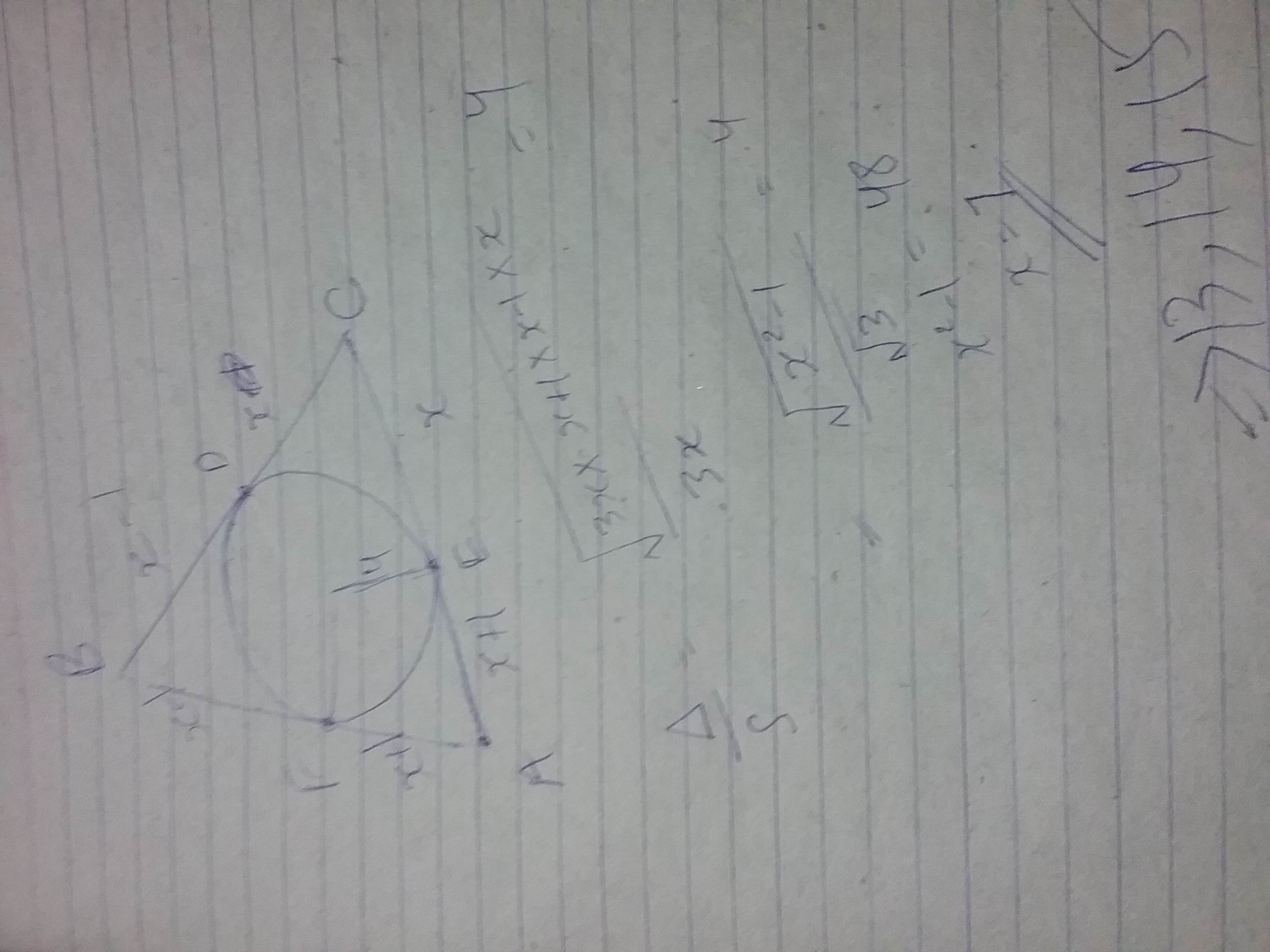- 4 years, 9 months ago

Another approach could have been by using the fact that in a triangle $tan\frac{A}{2} tan\frac{B}{2} +tan\frac{B}{2}tan\frac{C}{2} +tan\frac{C}{2} tan\frac{A}{2}= 1$

- 4 years, 9 months ago

- 4 years, 9 months ago

what did u do

- 4 years, 9 months ago

ab=bc=ca=8 root 3

- 4 years, 9 months ago

- 4 years, 9 months ago

Points?

- 4 years, 9 months ago

Wrong

- 4 years, 9 months ago

Problem 11, 5 marks

Find the number of polynomials of degree 5 with distinct coeffecients from the set {1,2,3,4,...,9} that are divisible by $x^{2}-x+1$

- 4 years, 9 months ago

1 hr and 15 minutes have passed

- 4 years, 9 months ago

It will be mid night after 6 hours :p

- 4 years, 9 months ago

See carefully the note again ;p

- 4 years, 9 months ago

As the problem is too hard to solve and going against rule 3,we are continuing with a new problem.

- 4 years, 9 months ago

It not that tough. I will upload solution in night. Its actually just simple forming of equation and then permutation-combination

- 4 years, 9 months ago

@Prince Loomba the answer is 288

- 4 years, 9 months ago

Nobody got right. @Ayush Rai give my points to me.

- 4 years, 9 months ago

Nobody gets the points as i have not mentioned it in the rules.You must also give the solution.

- 4 years, 9 months ago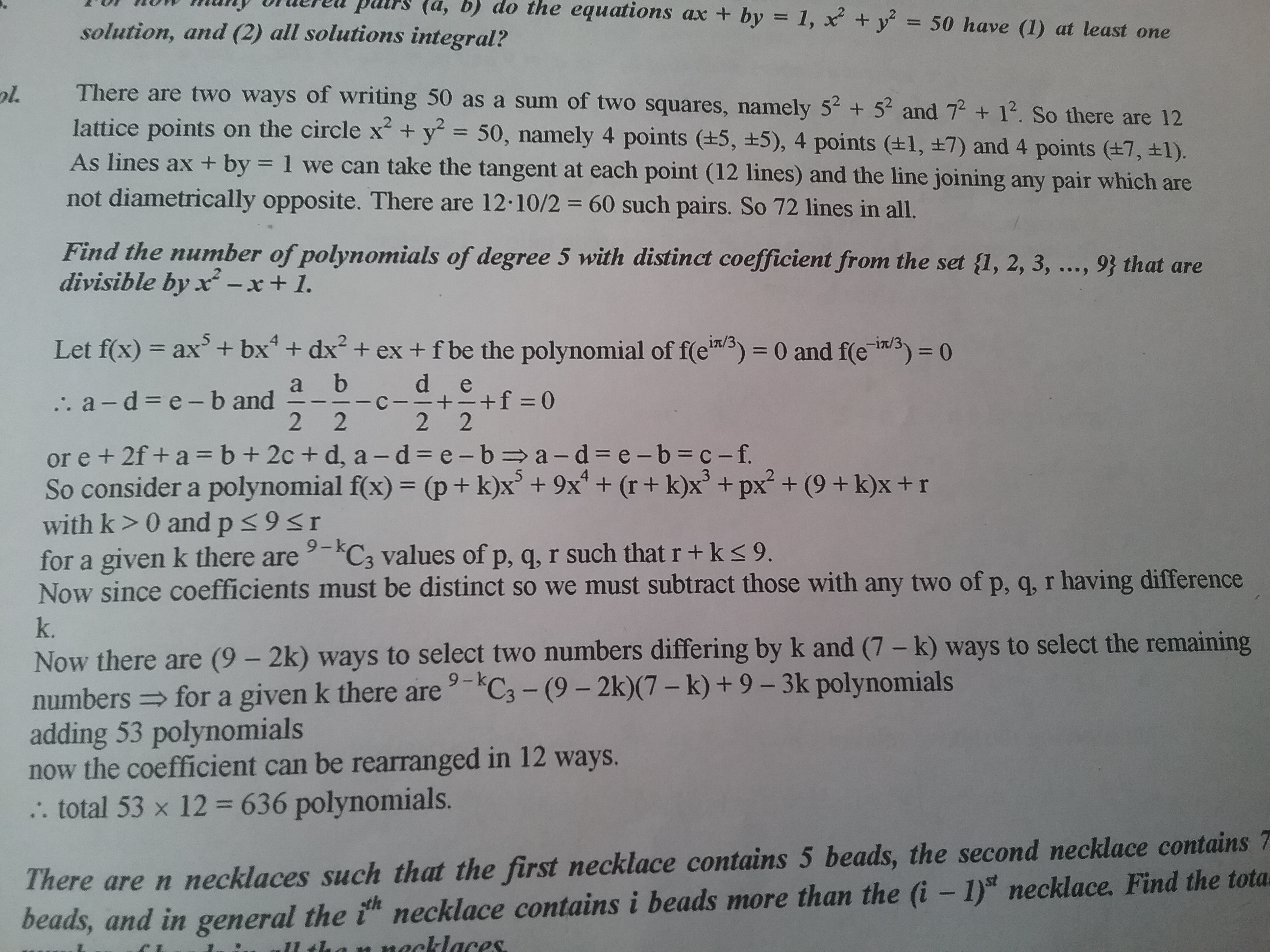- 4 years, 9 months ago

which book is this?

- 4 years, 9 months ago

Its FIITJEE 2012 RMO practice book

- 4 years, 9 months ago

u go to fiit jee

- 4 years, 9 months ago

No allen. My friend gave it to me.

- 4 years, 9 months ago

oh is it good?

- 4 years, 9 months ago

Very good. Talk in slack. Here its difficult to reply multiple times

- 4 years, 9 months ago

Let the polynomial $p(x)=a_5x^5+a_4x^4+a_3x^3+a_2x^2+a_1x+a_0.$
Then $(x^2-x+1)|p(x)\Rightarrow p(-w)=0.$
So,$a_5(1+w)+a_4(w)-a_3-a_2(1+w)-a_1w+a_0=0.$
$a_5-a_3-a_2+a_0=0\Rightarrow a_5+a_0=a_2+a_3$
$a_5+a_4=a_2+a_1.$Now we have to brute force according the condition.Well i just gave an idea.I am not so interested in combi as such.So i dont know the answer.

- 4 years, 9 months ago

Along the same lines I had a different idea: $p(\omega) = -a_{5}\omega ^2 + a_4 \omega - a_3 +a_2 \omega ^2 - a_1\omega + a_0 = 0$

Therefore,

$(a_2 - a_5)\omega ^2 + (a_4 - a_1)\omega + (a_0 - a_3) = 0$

Hence we get,

$a_2 - a_5 = a_4-a_1=a_0-a_3$

And similarly for $p(\omega ^2)$

- 4 years, 9 months ago

Bro 6 hrs passed i suppose

- 4 years, 9 months ago

PROBLEM 12 In an isosceles triangle ABC (AB = BC), N is the midpoint of the median BM and MD is perpendicular to CN (see the figure below). Prove that the angles BAD and ACN are equal.(2 MARKS)

- 4 years, 9 months ago

Where's the figure??

- 4 years, 9 months ago

Well sorry about that.Can u manage without that?

- 4 years, 9 months ago

Problem 13 : [3 marks]
If $a=\dfrac{b+c}{x-2},b=\dfrac{c+a}{y-2},c=\dfrac{a+b}{z-2},xy+yz+xz=67$ and $x+y+z=2010.$What is the value of $-xyz?$

- 4 years, 9 months ago

5892? Might be a calculating error Am I right?

- 4 years, 9 months ago

Yes you are correct.Now u must write the solution for it!

- 4 years, 9 months ago

First get that x-2=b+c/a -->x-1=(a+b+c)/a --->1/(X-1)=a/(a+b+c) Now get eqns for 1/(y-1) ,1/(z-1) Their sum is 1 Thus(x-1)(y-1)+(y-1)(z-1)+(x-1)(z-1)=(x-1)(y-1)(z-1 Now expand and put values to get answer

- 4 years, 9 months ago

Good one! Now post the next problem.

- 4 years, 9 months ago

Problem 14 : [3 marks]
$\dfrac{3}{1^2.2^2}+\dfrac{5}{2^2.3^2}+\dots+\dfrac{29}{14^2.15^2}=\dfrac{m}{n}.$Find the value of $m+n.$

- 4 years, 9 months ago

449

- 4 years, 9 months ago

You are absolutely correct.Give your solution and then post the next problem.

- 4 years, 9 months ago

It's general term is (2n+1)/n^2(n+1)^2 =((n+1)^2-n^2)/n^2(n+1)^2 =1/n^2-1/(n+1)^2 =1-1/15^2 =224/225

- 4 years, 9 months ago

correct solution..+1 post next problem with the points mentioned

- 4 years, 9 months ago

Problem 15:[1 marks]

Find the number of solutions to \begin{aligned} x+y+z&\geq150 \\ 0\leq x,y,z &\leq 60 \end{aligned}

- 4 years, 9 months ago

Are x,y,z integers?

- 4 years, 9 months ago

They should be

- 4 years, 9 months ago

x,y,z are integers.

- 4 years, 9 months ago

I am feeling too lazy to write the whole solution and even finding the correct answer. I will only share the idea that I had in mind. This question actually boils down to finding coefficient of $m^k$ ($k=x+y+z$) in the expansion of : $(1+m+m^2+m^3+\dots m^60)^3=(1-m^{61})^3(1-m)^{-3}$

I hope from here on its quite simple!

- 4 years, 9 months ago

There is a short soln also.

- 4 years, 9 months ago

From above condition we can get (60-x)+(60-y)+(60-z)<=30 Then introduce a dummy variable w and change the equation to (60-x)+(60-y)+(60-z)+w=30. Counting these cases is equal to counting the ways of distributing 30 apples in 4 people such that anyone can get any number of apples which are equal to 33C3.

- 4 years, 9 months ago

496

- 4 years, 9 months ago

No

- 4 years, 9 months ago

the area on which axis the x or the y

- 4 years, 9 months ago

I think graph itself is bounded as rectangles

- 4 years, 9 months ago

Problem 16 : [3 marks]
Given the sum :
$\dfrac{1}{1^4+1^2+1}+\dfrac{2}{2^4+2^2+1}+\dfrac{3}{3^4+3^2+1}+\dots$(upto infinity)$=\dfrac{a}{b}.$
What is the product $ab?$

- 4 years, 9 months ago

2? a=1 b=2

- 4 years, 9 months ago

- 4 years, 9 months ago

LaTeX: \begin{aligned} S & = \displaystyle \sum^{infty}_{n=1}\frac{n}{n^4+n^2+1} \\ & = \displaystyle \sum^{infty}_{n=1} \frac{n}{(n^2+1+n)(n^2+1-n)} \\ & = \frac{1}{2} \displaystyle \sum^{infty}_{n=1} \left(\frac{1}{n^2+1-n} - \frac{1}{n^2+1+n}\right) \\ & = \frac{1}{2} \left(1-\frac{1}{3}+\frac{1}{3} - \frac{1}{7} + \frac{1}{7} -\frac{1}{13} + \dots =1/2

- 4 years, 9 months ago

n^4+n^2+1=(n^2+n+1)(n^2-n+1) now convert it into telescoping sum to get answer

- 4 years, 9 months ago

Problem 17 : [ 4 marks] Prove that if integer a is not divisible by $5$ then $x^5-x-a$ cannot be factorised as product of two nonconstant polynomials with integer coefficients

- 4 years, 9 months ago

Firstly if it has roots, one has to be integral as conjugate complex root pairs are formed and , now to prove no integral root is possible, consider y as a root, take it to be 5k,5k+1..,5k+4

Break given equation...

y (y-1)(y+1)(y^2+1)=a, a is not multiple of 5

Then y=5k is eliminated as first term (y)

y=5k+1 and 5k+4 are eliminated by 2nd and 3rd terms respectively.

y=5k+2 and 5k+3 are eliminated by the last term, forming type 5m. Square them and add 1 to get the result.

Thus no y is possible.

So no real root. So cant be broken into factors, as one has to be linear if it can be broken ( the other has imaginary roots)

- 4 years, 9 months ago

wrong! it is possible that it has a quadratic factor and a cubic factor Pls give more details and explain more breifly

- 4 years, 9 months ago

Thats what I said. A real root must be there

- 4 years, 9 months ago

Your soln is wrong Suppose it can be factorised as (x2-3x+4)(x3+2) None of which has integer roots It isnt necessary to have an integer root

- 4 years, 9 months ago

Real root must be there, as your 2nd term has!

- 4 years, 9 months ago

Ok I got it now The soln is correct Though I had another soln in mind

- 4 years, 9 months ago

Finally somebody understood haha

- 4 years, 9 months ago

Now post prob 18 Be quick pls

- 4 years, 9 months ago

If or if and only if

- 4 years, 9 months ago

i think if is fine,

- 4 years, 9 months ago

Problem 18, 3 marks

Find all functions f such that:

$f (x-y)^{2}=f^{2}(x)-2xf (y)+y^{2}$

- 4 years, 9 months ago

Clarification: its $f ((x-y)^{2})$

- 4 years, 9 months ago

f(X)=X I dont think any other Is possible Am I right?

- 4 years, 9 months ago

Nope wrong

- 4 years, 9 months ago

First put x=y=0 then we have f(0)=((f(0)))^2 Thus f(0)=1 or f(0)=0 Now put x=y but they are not equal to zero then we have f(0)=(f(x))^2 -2x(f(x))+x^2 write rhs as (f(x)-x))^2 So if f(0)=0 Then f(x)=x Or else f(x)-x=1 f(x)-x=-1 Thus possible values of f(x) are x,x+1,x-1 Now put values of f(x) To see which satisfy and u will get the answer

- 4 years, 9 months ago

Right, exact solution!

- 4 years, 9 months ago

Attention: Since I dont have questions, and I requested Ayush to post them for me as he was angry as I posted from a book, and he said I was doing all this for points. So I am not participating anymore in this contest

I request Ayush to delete my points

- 4 years, 9 months ago

Problem 20 : [3 marks]
If $x=\dfrac{p}{q}$ where $p,q$ are integers having no common divisors other than $1$,satisfies
$\sqrt{x+\sqrt{x}}-\sqrt{x-\sqrt{x}}=\dfrac{3}{2}\sqrt{\dfrac{x}{x+\sqrt{x}}}$

- 4 years, 9 months ago

5/4??

- 4 years, 9 months ago

I meant 25/16

- 4 years, 9 months ago

Yes you are correct! Post your solution!

- 4 years, 9 months ago

- 4 years, 9 months ago

- 4 years, 9 months ago

Multiplying both sides by √(x+√x). Then after transposing and squaring, the result follows

- 4 years, 9 months ago

good one!

- 4 years, 9 months ago

Attention:
Problem 20 is the last problem of this contest.I will soon be conducting contest 2.Thank u for all those who have participated in this contest.

- 4 years, 9 months ago

So our winner of this contest-1 is @Kaustubh Miglani.Congratulations.I would also congratulate @Prince Loomba as he was in the lead but eventually had drop out due to some misunderstandings.

- 4 years, 9 months ago

Where are you in slack @Ayush Rai

- 4 years, 9 months ago

iam banned from slack

- 4 years, 9 months ago

why? Do u want ntse qp

- 4 years, 9 months ago

yup.give me.

- 4 years, 9 months ago

how?

- 4 years, 9 months ago

- 4 years, 9 months ago

- 4 years, 9 months ago

@Ayush Rai check generaldiscussions.

- 4 years, 8 months ago

@Ayush Rai check generaldiscussions.

- 4 years, 8 months ago

dont leave space. between the brackets

- 4 years, 9 months ago

how?

- 4 years, 9 months ago

its a very long story.cant explain it to u now.preparing for NTSE

- 4 years, 8 months ago

ok so when are u going to be back

- 4 years, 8 months ago

back from where? iam banned from lounge

- 4 years, 8 months ago

OMG this is not good

will miss you

- 4 years, 8 months ago

can u give ur email

- 4 years, 8 months ago

raiayush234@gmail.com

- 4 years, 8 months ago

- 4 years, 8 months ago

@Ayush Rai. do not fail to reply . Just say a goodbye atleast

- 4 years, 8 months ago

what? after soooooo much motivation still u are leaving. U are good in biology better than all of us. Why feel inferior? Fight for urself.Hope u change ur mind.

- 4 years, 8 months ago

Its not that. You see there r very few who speak bio there. At times I feel mistreated too @Ayush Rai

- 4 years, 8 months ago

ok then may god bless u and hope u have a bright future.

- 4 years, 8 months ago

What do u mean. Anyways thanks for blessing. I think I need some more time to think over it @Ayush Rai and @Anik Mandal

- 4 years, 8 months ago

are u here @Ayush Rai ???

- 4 years, 8 months ago

Good bye @Ayush Rai and @Anik Mandal till I am ready for slack. THANK U for being good to me.

- 4 years, 8 months ago

Just see me I am good at nothing but I am present there . Hahahah I think you can contribute in biology there. No needto deactivate your account.

- 4 years, 8 months ago

hmm. How did you know that I have left slack? @Rohit Camfar

- 4 years, 8 months ago

- 4 years, 8 months ago

I know that . I remember you very well. did anyone say to u that I have left?

- 4 years, 8 months ago

Nope I saw your account deactivated

- 4 years, 8 months ago

ok @Rohit Camfar good

- 4 years, 8 months ago

Why good?

- 4 years, 8 months ago

just like that Anyways goodbye (from slack)

- 4 years, 8 months ago

- 4 years, 8 months ago

14 will b 15 on JAN 7 Why did u ask

- 4 years, 8 months ago

when I saw Vishwathiga Biotechnologist I thought you too be a professional whose age should be between 30 and 40

- 4 years, 8 months ago

hahaha no never. It is my dream.(biotech)

- 4 years, 8 months ago

ok are you leaving brilliant or slack

- 4 years, 8 months ago

slack only why?

- 4 years, 8 months ago

we tried our best rest upon u. But rememberif you leave slack hen not because ur not capabe to keep with physics maths chemistry but because you don't want to. Think from within your heart.

- 4 years, 8 months ago

b'bye I am making this discussion slack. Could be scolded by Calvin.

- 4 years, 8 months ago

bye

- 4 years, 8 months ago

Why are you leaving?@vishwathiga jayasankar

- 4 years, 8 months ago

I dunno anik ji. I feel inferior and ignored there. No matter haw hard I try to console myself. I just cant take all anymore. I am not as good in math and physics like you guys. This makes me feel like I have got nothing to do there @Anik Mandal I just dont fit in there. Please do reply.

- 4 years, 8 months ago

Always learn to take the positives.Someone is good in some thing others are good at some other thing...But don't feel demotivated...Start from small steps...Soon you will make giant strides.Believe in yourself!

- 4 years, 8 months ago

@Ayush Rai You posted the same question there ... Told told me it is from junio r ramanujan contest.

- 4 years, 8 months ago

If you found the circumradius of the triangle then use the property that the centroid of a triangle divides the median ib the ratio two is to one .No need to do such a long calculation.

- 4 years, 8 months ago

Thanks for the suggestion

- 4 years, 8 months ago

Hello

- 4 years, 6 months ago

do u want something?

- 4 years, 6 months ago

solution since abc dbc are equilateral triangles with the same base there is only one possibility that is both the triangles super impose on each other .so A =D.we know that BD=CD because equilateral triangle and BD is the radius so CD is also the radius. choose a point p ,such that it is perpendicular to AC or rather it is the extention of AC radius. now even PA also be the radius as the point p is on the circumference and a is the centre. join PB. since they are radius AC= BA and PA=AB (radius)since we have choosen the point p as the extention of AC and they are perpendicular .in triangle PBD PD=BD, and PAD =90 . so it is right angled triangle. hence proved.

- 4 years, 9 months ago

You are wrong.The points A and D do not coincide..

- 4 years, 9 months ago

Problem 5(4 marks)

Five identical empty buckets of 2-liter capacity stand at the vertices of a regular pentagon. Cinderella and her wicked Stepmother go through a sequence of rounds: At the beginning of every round, the Stepmother takes one liter of water from the nearby river and distributes it arbitrarily over the five buckets. Then Cinderella chooses a pair of neighboring buckets, empties them into the river, and puts them back. Then the next round begins. The Stepmother’s goal is to make one of these buckets overflow. Cinderella’s goal is to prevent this. Can the wicked Stepmother enforce a bucket overflow?

- 4 years, 9 months ago

i think no

- 4 years, 9 months ago

Problem 7 (1 mark)

Prove that for any acute triangle, $a^{2}+b^{2}>c^{2}$, where c is the longest side.

- 4 years, 9 months ago

Directly use cosine rule and put cos>0 so simple! :)

- 4 years, 9 months ago

Right

- 4 years, 9 months ago

But you And abhishek posted simultaneously

- 4 years, 9 months ago